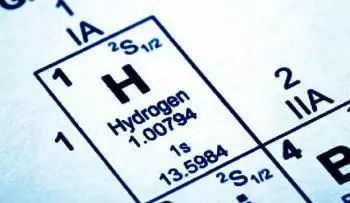# What Is the Atomic Number of an Element?

The atomic number indicates the total number of protons in the nucleus of a given atom. We represent it with the letter Z. The number is placed as a subscript to the left of the element symbol.

What you need to know to calculate ...
What you need to know to calculate the number of electrons or neutrons in an atomAtoms are made up of a nucleus, and a shell made up of electrons. The nucleus is made up of two subatomic particles: protons and neutrons. Protons are positively charged, neutrons are electrically neutral. Therefore the atomic nucleus always has a positive charge.

On the other hand, electrons have a negative charge.

An atom in its natural state is neutral. In this case, a neutral atom has the same number of electrons and protons.

The atomic number alone that determines the chemical properties of an element

The total weight of an atom is called the atomic weight. It is approximately equal to the number of protons and neutrons, with a little extra added by the electrons.

## What Is the Role of the Periodic Table in the Atomic Number Definition?

The atomic number is used to classify the elements within the periodic table of the elements. The periodic table is an arrangement in which their atomic number arranges chemical elements.

Each chemical element in the periodic table is characterized by having the same number of protons in its atomic nucleus. However, the same chemical element can have a different number of neutrons (N).

In 1911, Ernest Rutherford gave a model of the atom. In this model, the nucleus held most of the atom's mass and a positive charge. According to him, it was similar to half of the atom's atomic weight, expressed in numbers of hydrogen atoms.

## What Are Isotopes and the Mass Number?

Isotopes are chemical elements that have the same number of protons (Z) but different numbers of neutrons (N). The chemical properties of isotopes are very different in some cases. Around 75% of naturally occurring elements exist as a mixture of isotopes.

The mass number (A) of an atom is the sum of the atomic number (Z) and the number of neutrons (N).

## How Has the Atomic Number Evolved Throughout History?

At first, the atomic number was the position in which a chemical element remained when they were arranged in increasing order according to their atomic masses.

In 1913, Johannes H. van den Broek discovered that the number of elementary charges in the atomic nucleus was equal to the atomic number. Later, Niels Bohr adopted this discovery to develop his quantum theory about the structure of atoms and the origin of spectra. With this theory, he reflected in the well-known Bohr atomic model.

Author:

Published: May 20, 2019
Last review: April 27, 2021